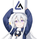3865 görüntülenme
The new array feature is extremely powerful, as it will allow pinescript users to do more complex things, or compute existing calculations more efficiently, it will also be possible to shine some light to some already existing functions, one of them being percentile_nearest_rank.

We have been working on this new feature with our pal alexgrover, and made this script which computes a rolling percentile using the nearest rank method.

Settings

• Length: Window of the rolling percentile, determine the number of past data to be used.
• Percentage: Return the current value if Percentage% of the data fall below that value, the setting is in a range (0,100).
• Src: Input source of the indicator.

Usage

A rolling percentile can have many usages when it comes to technical analysis , this is due to its ability to return the value of three common rolling statistics, the rolling median, which can be obtained using a percentage equal to 50, the rolling maximum, obtained with a percentage equal to 100, and the rolling minimum, obtained with a percentage equal to 0.

When we use our rolling percentile as a rolling median, we can obtain a robust estimation of the underlying trend in the price, while using it as a rolling maximum/minimum can allow us to determine if the market is trending, and at which direction. The rolling maximum/minimum is a rolling statistic used to calculate the well known stochastic oscillator and Donchian channel indicator.

We can also compute rolling quartiles, which can be obtained using a percentage of 25 or 75, with one of 25 returning the lower quartile and 75 the upper quartile.

In blue the upper rolling quartile (%75), in orange the lower rolling quartile (%25), both using a window size of 100.

Details

In order to compute a rolling percentile nearest rank, we must first take the most recent length closing prices, then order them in ascending order, we then return the value of the ordered observations at index (percentage/100*length) - 1 (we use - 1 because our array index starts at 0).